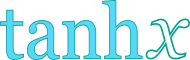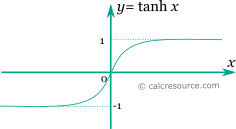Hyperbolic tangent calculator

This tool evaluates the hyperbolic tangent of a number: tanh(x). Enter the argument x below.

 x = Result: tanh(x) =Definitions

General

The hyperbolic tangent function is defined as:

The graph of the hyperbolic tangent function is shown in the figure below. It is a monotonic function unlike the trigonometric tangent, which is periodic .Series

All hyperbolic functions can be defined in an infinite series form. Hyperbolic tangent function can be written as:

The above series converges for . Bndenotes the n-th Bernulli number.

From the expanded form of the series it can be seen that the higher terms become insignificant, for values of x close to zero, resulting in the following quite useful approximation:

Properties

The derivative of the hyperbolic tangent function is:

The integral of the hyperbolic tangent is given by: